# Physics - Classical Mechanics - Conservation of Momentum[Image 1]

## Introduction

Hey it's a me again @drifter1! Today we continue with Physics and more specifically the branch "Classical Mechanics" to start talking about Momentum. So, without further ado, let's get straight into it!

## Momentum

Talking about moving objects, momentum is equal to the product of the mass of the object and its velocity. It's a vector quantity (has magnitude and direction) with the same direction as the velocity. Based on Newton's second law of motion the change in momentum is equal to the force that acts on an object. But, what exactly that this mean for us? Well, momentum is the quantity that shows us how much is moving (mass) and how fast it moves (velocity). It can be defined as "mass in motion" meaning that it somewhat shows us how hard (how much force) we have to apply to stop an object.

Mathematically, we write momentum as: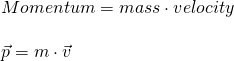The symbol that is used to describe momentum is the lower case 'p'. From the quantities that take place in the previous equation we understand that the S.I. unit of momentum is kg ·m/s.

Newton's 2nd law looks like this: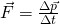Knowing that the mass is a constant and that the change of velocity over time is equal to the quantity known as acceleration (a), we can write the commonly known 2nd law equation: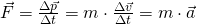### Example

Determine the momentum in the following cases:
1. 80-kg person moving at 3 m/s
2. 2000-kg car moving at 30m/s
3. 40-kg kid running at 5 m/s
1.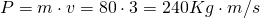2.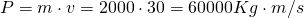3.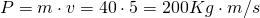Note: You can see that the kid has a smaller momentum, even though its faster than the person, because it has less mass and so a smaller product.

## Conservation of Momentum

An important and very powerful law in Physics is the "law of momentum conservation". Based on Newton's third law (each action has a reaction - the forces are equal in magnitude and opposite in direction), in collisions between two objects, the momentum changes are equal in magnitude and opposite in direction, conserving the total momentum of the isolated system.

Mathematically, the conservation looks like this: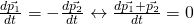This equation contains the momentum p1 of an object 1 and the momentum p2 of an object 2. Supposing that these objects are in an isolated system, a collision between object 1 and object 2 would cause an equal in magnitude but opposite in direction change in momentum, therefore keeping the total momentum of the system (p1 + p2) constant.

This of course applies to any number of objects, which are in an isolated system. Generalizing it, for any initial and any final state of such an system and N objects, we can write:
Various types of collisions with examples and other very interesting topics around momentum and its conservation will be covered in follow-up articles!

## RESOURCES:

### References

1. https://www.britannica.com/science/momentum
2. https://www.physicsclassroom.com/Class/momentum/u4l1a.cfm
3. https://www.physicsclassroom.com/class/momentum/Lesson-2/Momentum-Conservation-Principle
4. http://scienceworld.wolfram.com/physics/ConservationofMomentum.html

### Images

1.## Final words | Next up

This is actually it for today's post! Next time we will get into Collisions...

See ya!Keep on drifting!

H2
H3
H4
3 columns
2 columns
1 column